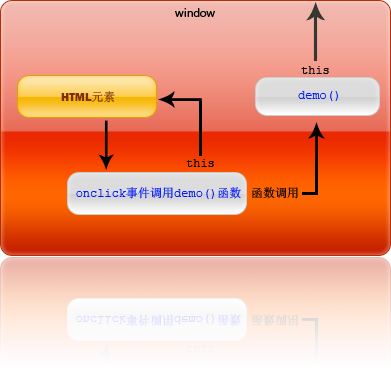# 你不知道的 JavaScript - “this”

JavaScript 里的 this 到底指得是什么？很多人都会告诉你 this 指的是当前对象。这样理解对么？在大多数情况下确实没错。比如我们经常会在网页上写这样的 JavaScript：<input type="submit" value="提交" onclick="this.value='正在提交数据'" />var foo = function() {console.log(
this);}foo();new foo();<input type="button" id="aButton" value="demo" onclick="" /><script type="text/javascript">function demo() {this.value = Math.random();}</script>document.getElementById("aButton").onclick = demo;<input type="button" id="aButton" value="demo" onclick="demo()" /><input type="button" id="aButton" value="demo" /><script type="text/javascript">var button = document.getElementById("aButton");function demo() {this.value = Math.random();}button.onclick
= demo;alert(button.onclick);</script>function demo() {this.value = Math.random();}<input type="button" id="aButton" value="demo" onclick="demo()" /><script type="text/javascript">var button = document.getElementById("aButton");function demo() {this.value = Math.random();}alert(button.onclick);</script>function onclick() {demo();}<script type="text/javascript">function demo(obj) {obj.value
= Math.random();}</script><input type="button" value="demo" onclick="demo(this)" /><input type="button" value="demo" onclick="demo(this)" /><input type="button" value="demo" onclick="demo(this)" />function new (somefunction) {var args = [].slice.call(arguments, 1);somefunction.prototype.constructor
= somefunction;somefunction.apply(somefunction.prototype, args);return somefunction.prototype;}

5
0